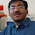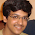## Feb 6, 2011

Source: Posted by chera (Gaurav Sinha, IITK 1996 Graduate, Indian Revenue Service) in comments on Consecutive Heads Problem

Problem:
Suppose you have a fair coin and you toss it until you have got equal number of heads and tails. What is the expected number of tosses? Note that probability that the game stops in odd number of tosses is 0. The probability that the game stops in 2 tosses = 0.5

Solution:
Different solutions posted by Kalyan Parhi (EE IITB Alumnus), Abhash, Siva, Gaurav Sinha (CSE IITK 1996 Alumnus, Indian Revenue Service), Dinesh Krithivasan (IITM Alumnus, Phd University of Michigan, Senior Qualcomm Engineer) in comments!

1.E(X)=sum_(1 to inf) binomial(2n,n)*2^(-2n).
now as n->inf by stirling approximation T_n->n^(-.5)
which, by cauchy convergence criterion, is divergent.
I dunno what's wrong.

2.sorry about previous argument..In E(X) nth term T_n has probabilty = p_n = q_(2n-2)-q_2n..where q_2n=binomial(2n,n)*2^(-2n)..solving E(X)=2*p_1+4*p_2+...=2(1-q_2)+4(q_2-q_4)+...=2(1+q_2+q_4...)..now we can show as from previous comment that this diverges..so still clueless..:(

3.The expected number diverges for me too.

For all the situations where the absolute difference between the number of heads and tails is same the expected number of tosses required to reach the situation of equal number of heads and tails is same. Also, if the current difference is 'd' then with equal probability the difference may increase or decrease after the next toss. Let E(d) be the expected number of tosses required to get equal number of heads and tails when the current difference between their counts is d. So, E(d) = 1 + 0.5 * E(d-1) + E(d+1). Also, E = 1 + E(1). and E(0) = 0. Solving this, E = i + (1/i)*E(i). Since for any i E(i) will be positive. The result diverges.

4.This is a problem involving catalan numbers..

E(x) = 2*sum 2n*C(2n-2)* 1/2^2n which is indeed divergent.

The reason is that the we are dealing with a random walk. There is a good chance (1/2) in this case that the number of heads are never equal to the number of tails. The proof is fairly trivial using recursion.

For a general coin which is not fair, the probability that the number of heads is ever equal to the number of tails is min(p,1-p). again as I said the proof is trivial by recursion.

5.@kalyan.. Yes, it diverges. But I think T_n->n^(0.5) and not n^(-0.5). Please check if I am wrong!

@abhash.. very interesting approach..

Let E(d) be the expected number of tosses required to get equal number of heads and tails when the current difference between their counts is d.

E(d)= 0.5(1+E(d+1)) + 0.5(1+E(d-1)) for d>1
i.e.

E(d) = 1 + 0.5*(E(d-1)+E(d+1)) for d>1
i.e.
E(d-1)=2*E(d)-E(d+1)-1 for d>1
and E(1) = 1+0.5*E(2)
E(0)=0

We need to find the value of E: E=0.5(1+E(1))*2=1+E(1)

@abhash: How did you solve this recurrence?

6.@kalyan.. Sorry. Got my formula for stirling approximation wrong. You are correct! It diverges as T_n->n^(-.5)

7.the probability that eventually H=T is one,i.e. almost certain.

and in a general case of biased coin, where the probability of head is p, probability that eventually H=T is 2*min(p,1-p).

Proof is available at math.stackexchange.com/questions/17999/1d-random-walk-probability-to-go-back-to-origin.

8.@chera.. Thanks a ton!
@Siva.. I take my statement about your comment back. Your probability is not correct, and hence your solution (both the calculation and the approach) is wrong!

9.Yes of course.. I take my comment back... I missed a factor of 2.
so instead of 2*min(p,1-p) I wrote min(p,1-p) which led to to the wrong answer. I wish I could edit my post.

10.a solution for finding probability that eventually heads=tails in case of a biased coin.

consider a random walk with probability p of moving right and 1-p of moving left. u start at origin O. assume wlog that p<1/2. suppose the probability that eventually u return to origin is x. there are two possibilities:

Event E1: first step is towards right. In this case,we can assume that certainly u will return to origin as p<1/2. so probability(E1)=p.

Event E2: first step is towards left to point A, and u return to origin O after crossing A n times.
probability (E2 for a fixed n)=(1-p)(p)(x-p)^n.

hence x= probability(E1)+probability (E2)
i.e. x=p+ sigma (1-p)(p)(x-p)^n.
where sigma is from n=0 to infinity.

on summation of geometric series and simplification, we get quadratic equation
(x-1)(x-2p)=0.

so x=2p ignoring x=1.

as we assumed p<1/2,
general solution is x= 2 min(p,1-p)

11.Edit to my first post:
This is a problem involving catalan numbers..(http://en.wikipedia.org/wiki/Catalan_number)
I think my earlier expression for expectation is most probably correct.

earlier poster makes no mention of the expectation which is what the question asks for.

This expectation is infinite and can also be explained as a direct consequence of the optional stopping theorem
(http://en.wikipedia.org/wiki/Optional_stopping_theorem)

12.@pratik, To solve the recurrence find the value of E(d) in terms of the E(d+1). It turns out to be

E(d) = d + d/(d+1) * E(d+1)

This can be done using induction. If we plugin this to find the value of E we get,

E = d + 1/d * E(d)

This result is true for any d > 1 and can be proved via induction.

13.A (standard) generating function approach. Let P(x) = sum_{n=0}^{\infty} p_n x^n where p_n is the probability that the walk returns to origin in 2n steps. note that p_0 = 1. Similarly, let Q(x) = sum_0^{\infty} q_n x^n where q_n is the probability that the walk returns to the origin for the first time in 2n steps. Note that q_0 = 0. Then,

p_n = \sum_{k=1}^{n} q_k p_{n-k}

The convolution suggests going to the generating function domain where this equation becomes P(x) - 1 = P(x)Q(x) or Q(x) = 1-(1/P(x)). Its easy to see that p_n = binomial(2n,n)(pq)^n. The generating function for the central binomial coefficient is 1/sqrt(1-4x). So Q(x) becomes 1-sqrt(1-4pqx). Q(1) gives the probability of return at some point to the origin and can be seen to equal 1-|p-q|. So probability of the walk escaping to infinity is |p-q|. If the walk isn't symmetric, then immediately the expected time of first return in infinite. If p=q, the expected time of first return is the derivative of 1-\sqrt{1-x} at x=1 which is again infinite. By extracting coefficients out of Q(x) using binomial series, we can compute q_n as well.

14.The probability that if we had 2k trials, we'll have equal heads and tails:
\binomial(2k,k)*2^(-2k)

Therefore, expected value is
\sum_{K=1}^{K=\infty} 2k*\binomial(2k,k)*2^(-2k)

15.@Sumit, we also have to ensure that in first 2r trials H never equals T, where r<k.

If there are 2k trials, total number of ways will be 2C(k-1) where C(n)= binomial(2n,n)/(n+1) is well known as catalan number.

1.@chera , How did you get the total number of ways for 2k trials ?

### Fraction Brainteaser

Source: Sent to me by Gaurav Sinha Problem: Siddhant writes a Maths test and correctly answers 5 out of 6 Arithmetic questions and 20...